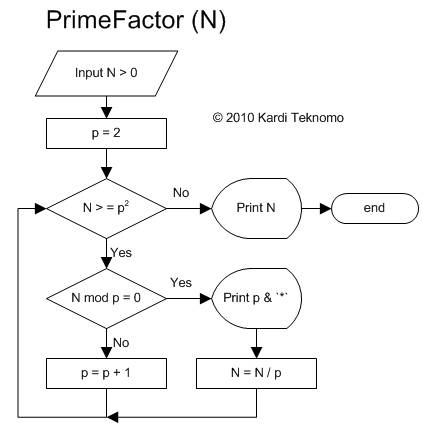## Algorithm for Prime Factorization

Now we proceed with algorithm (method) to compute prime factor manually by hand computation.

The simplest algorithm to find the prime-factor is by repeatedly dividing the number with the prime factor until the number becomes 1.

Suppose our number is 100

We find smallest prime number that actually divides 100 and we found 2

Thus 100 divided by 2 become 50. Now our number becomes 50.

We find smallest prime number that actually divides 50 and again we found 2

Thus 50 divided by 2 become 25. Now our number is 25.

We find smallest prime number that actually divides 25 and we found 5 .

We divide 25 with 5 and produces 5. Now our number is 5.

Since 5 is also a prime number, our smallest prime number is 5 .

The division of 5 with 5 produces 1 and we stop the computation and gathering the result such that 100 = 2 x 2 x 5 x 5

When we use procedural programming, the algorithm of prime factor is as follow

Input N p = 2 Print N & "=" Do while N > = p * p If N mod p = 0 then Print p & "*" N = N / p (divide by prime number) Else p = p + 1 End If Loop Print N

The flow chart of above algorithm is shown below.After learning about divisors, prime and composite number as well as prime factorization, you may want to try the following online Divisibility-Prime-Factorization calculator. This interaction program below determines whether your input is a prime or composite number. If it is a composite number, the program will list the divisors and gives the prime factorization which is unique for your input number.

Input any positive integer number larger than 1:

Result:

In the next section, we will discuss slightly more challenging problem: how to calculate prime factor of any positive integer in Spreadsheet without macro (without do while loop). You need to think to do it in parallel programming.

< Previous | Next | Content >